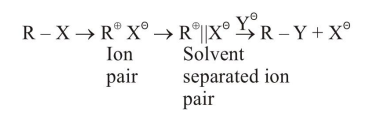# The mechanism of

Question:

The mechanism of $S_{N}{ }^{1}$ reaction is given as :A student writes general characteristics based on the given mechanism as :

(a) The reaction is favoured by weak nucleophiles

(b) $R^{\oplus}$ would be easily formed if the substituents are bulky

(c) The reaction is accompained by recemization

(d) The reaction is favoured by non-polar solvents.

Which observations are correct ?

1. b and d

2. $\mathrm{a}$ and $\mathrm{c}$

3. $a, b$ and $c$

4. $\mathrm{a}$ and $\mathrm{b}$

Correct Option: , 2

Solution:

$\mathrm{S}_{\mathrm{N}}^{1}$ favours

(a) The reaction is favoured by weak nucleophiles

(b) $\mathrm{R}^{\oplus}$ would be easily formed if the substituents are bulky

(c) The reaction is accompained by recemization